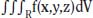NextPrevious

# Are there double and triple integrals?

Yes, there are double and triple integrals—and even multiple integrals in equations (∫∫, ∫∫∫, and so on). For example, the integration of a function of three variables, w = f(x, y, z), over a three-dimensional region R in xyz-space (three-dimensional space) is called a triple integral. The notation is as follows:To compute the iterated integral, we need to integrate with respect to z first, then y, then x. And when we integrate with respect to one variable, all the other variables are assumed to be constant.

Close

This is a web preview of the "The Handy Math Answer Book" app. Many features only work on your mobile device. If you like what you see, we hope you will consider buying. Get the App